## 1. Street Sideline Intersection

 Two intersecting streets are shown on the right. The centerline direction for each is known as are their widths. Given the coordinates of a point on each centerline, what are the coordinates of the sideline intersection at point P?Since bearings are given, this is a bearing-bearing intersection. Because point P is not on the centerlines it should not be connected directly to the two given coordinate points. Instead, create two new points, G and H, on each sideline. These will serve as the baseline for the intersection triangle.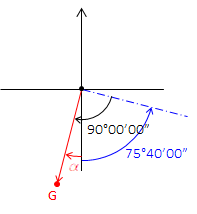To create point G, go perpendicular to the centerline at the given coordinate: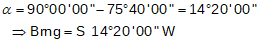Do the same to create point H:Inverse to determine the length and direction of the baseline GH:Sidelines have the same directions as their respective centerlines. Using those, compute the three angles:Then using the Law of Sines, determine the two missing sides: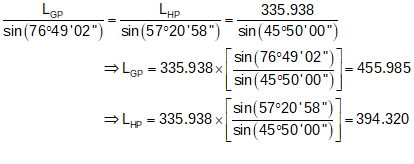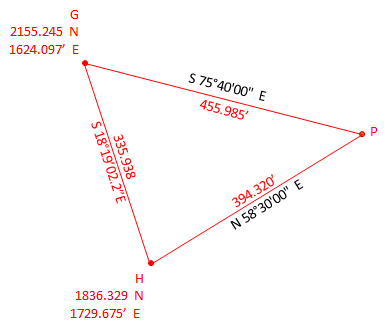Compute point P from point G:Math check - compute point P from point H: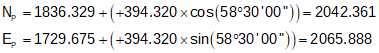check

## 2. Intersecting Curves

### a. Street Lines

 Coordinates of point A are 2000.00' N and 500.00' E. Using the curve geometry shown, compute coordinates of the labeled points.Because sine and cosine of Azimuths will always return the correct mathematical sign in coordinate computations, they will be used instead of Bearings. Starting at point A, compute azimuths to the side points and all the way to point CSide points G and D at A.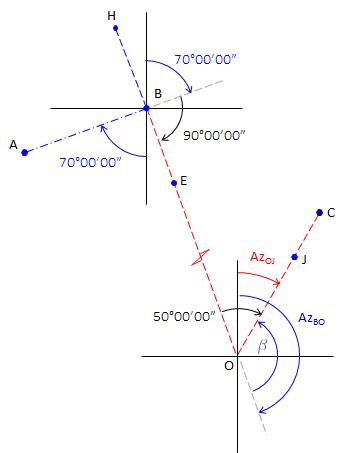Side points H and E at B; Continue to center of arc then cul-de-sac center.Compute sideline points D and G coordinates from point A:Compute point B from point A:Then the sideline points H and E from B: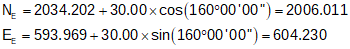Starting at point B, compute points O, J, and C:Point F cannot be directly computed from a known point. Because its position is defined by the intersection of the southerly street sideline and the cul-de-sac, it is connected by radii to points O and C. Its position is computed from a distance-distance intersectionWe have the length and direction of the baseline OC. We also have lengths of the other two sides of the intersection triangle: dCF = 60.00' and dOF = 300.00'-30.00' = 270.00' Because a distance-distance intersection results in two possible point locations, we must take care to solve the correct triangle.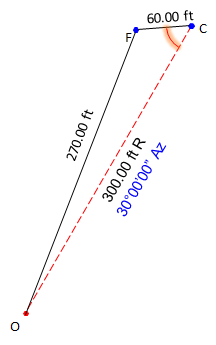Use Law of Cosines to solve angle at C:Then the direction from C to F:And finally the coordinates from point C:Similarly, point I is at the intersection of the northerly street sideline and the cul-de-sac. Intersection traingle sides are 300.00', 60.00' and 330.00'. As before, there are two possible intersection locations. After determining correct triangle to solve:Use Law of Cosines to solve angle at C:Then the direction from C to I:And finally the coordinates from point C:Coordinates Summary:### b. Curvilinear Traverse

Lets add a lot and compute its coordinates and area.Starting at point C, compute points Q, R, and S. Convert bearings to azimuhts for ease of computations.Point T must be computed by intersecting the westerly lot line with the southerly street sideline. Point T is connected to point S by a bearing and point O by the southerly curve radius.Line SO will serve as the baseline for the intersection triangle. Compute its length and direction from point S's and O's coordinates.Compute angle at S from directions of SO and ST:Use Law of Sines to solve angle at T:(since the angle at T is less than 90°, this is the correct angle). Compute angle at O:And length of line ST:Finally, compute point T from point S:To determine the Lot area, we will use the coordinate method then take into account the segment areas caused by the two arcs. To compute the segment areas we need the central angle for each arc.

 The following equations come from the VII COGO topic. Segment area:(Eqn F-7) Central angle:(Eqn F-3)

Coordinate method area:Arc TF:Arc FQ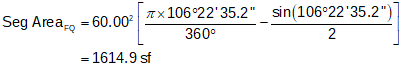Total Lot area:Final Lot dimensions:The North and East coordinates of each alignment point are:The Total Station Instrument (TSI) is set up at a known point from which part of the alignment will be staked. Using the coordinates, the distance and direction from the TSI point to each alignment point. is computed. After orienting the TSI on a known basksight (BS) point, an alignment point is staked by turning to its azimuth and measuring out the distance.

Example computations:

 Set TSI at point B. Sight point A with AzB-A=255°42'45" set on the horizontal circle.To stake 10+88.40 BCTurn to horizontal angle reading = 238°25'40" Stake point at 227.17 ft
 To stake 11+00Turn to horizontal angle reading = 235°42'10" Stake point at 222.69 ft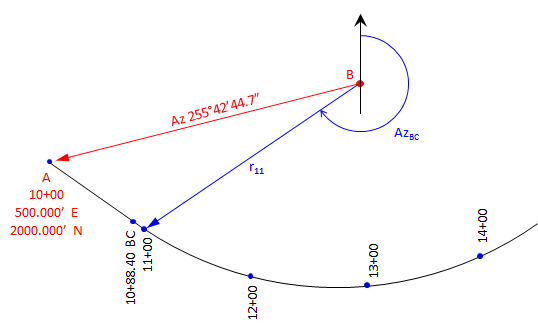To stake 12+00Turn to horizontal angle reading = 209°17'17" Stake point at 189.79 ftand so on for as many points to be staked from point B.

 Move the TSI to point C; Sight point B with AzC-B = 303°54'34" set on horizontal circle.To stake 14+00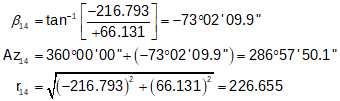Turn to horizontal angle reading = 286°57'50" Stake point at 226.66 ft
 To stake 14+56.98 EC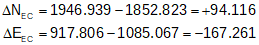Turn to horizontal angle reading = 300°08'26" Stake point at 193.411 ft
 To stake 14+96.29 BC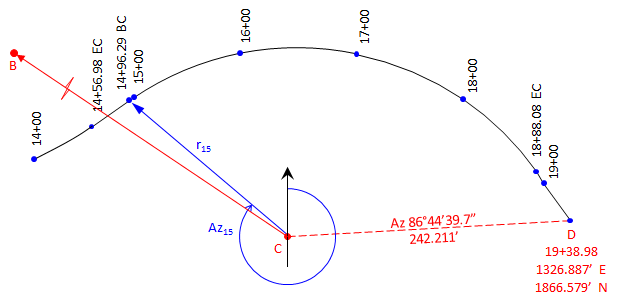Turn to horizontal angle reading = 310°48'54" Stake point at 178.41 ft

Depending on sight distances, obstructions, etc, the TSI may need to be relocated a few times to stake all the points.

Since radial stakeout is a series of open traverses, there should be overlap between different TSI setups as field checks. For example, stations 13+00 through 15+00 should be computed from points B and C: they would be staked from one TSI set up and checked from the other.

A complete set of stakeout notes would be:

 TSI at point B; BS point A Point Az, Azimuth r, Distance A - BS 255°42'45" - - 10+88.40 BC 238°25'41" 227.17 11+00 235°42'10" 222.69 12+00 209°17'17" 189.79 13+00 177°53'54" 173.67 14+00 145°21'03" 180.72 14+56.98 EC 128°23'50" 194.30 14+96.29 BC 118°01'09" 209.01 15+00 117°07'24" 210.75 C - Check 123°53'34" - -

 TSI at point C; BS point B Point Az, Azimuth r, Distance B - BS 303°54'34" - - 13+00 286°57'50" 226.66 14+00 299°21'58" 191.22 14+56.98 EC 310°48'54" 178.41 14+96.29 BC 311 58'29 177.50 15+00 345 36'34 161.96 16+00 20 42'10 161.12 17+00 52 04'50" 190.59 18+00 75 23'29" 219.64 18+88.08 EC 78 14'51" 224.09 19+00 86 44'39" 242.21 D - Check 86°44'40" - -
Hits: 200